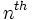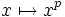# Difference between revisions of "Perfect (mathematics)"

## Contents

### In group theory

Perfect group: A group that equals its own commutator subgroup.

### In topology

Perfect space: A topological space where every point is closed, and is an intersection of countably many open subsets containing it.

Perfectly normal space: A normal space where every closed subset is an intersection of countably many open subsets containing it.

Perfect set: A set in a metric space that has no isolated points.

### In number theory

Perfect power, for instance, perfect square or perfect cube: A perfectpower is an integer that occurs as thepower of an integer.

Perfect number: A natural number that equals the sum of all its proper divisors.

### In field theory

Perfect field: A field that either has characteristic zero, or hasandis a surjective map.

### In graph theory

Perfect graph: A graph with the property that for every induced subgraph, the chromatic number equals the clique number.

Perfect matching: A matching in a bipartite graph such that every element on one side gets matched to exactly one element on the other side.